# What Is The Pythagorean Theorem? (5 Key Things You Should Know)

The Pythagorean Theorem is used often in geometry & trigonometry when we study right triangles.  It helps us to solve right triangles when a side length is missing.

So, what is the Pythagorean Theorem?  The Pythagorean Theorem relates a right triangle’s sides by the equation a2 + b2 = c2, where a and b are the legs and c is the hypotenuse. It cannot be used by itself to find angles, and it only works for right triangles. The Law of Cosines is the more general rule to find angles in triangles.

Of course, knowing the most common Pythagorean Triples (sets of 3 numbers that satisfy a2 + b2 = c2) will help us to recognize right triangles.

In this article, we’ll talk about the Pythagorean Theorem, when to use it, and how to apply it.  We’ll also answer a few other common questions about the Pythagorean Theorem.

Let’s get started.

## What Is The Pythagorean Theorem?

The Pythagorean Theorem relates the three sides of a right triangle by the following equation:

• a2 + b2 = c2

where a and b are the legs (the short sides) of the triangle and c is the hypotenuse (the long side, which is across from the 90 degree angle).

The Pythagorean Theorem is sometimes expressed in terms of the areas of squares.  Specifically, the area of a square with side length c is equal to the sum of the areas of two squares with side lengths a and b.

You can see this concept illustrated in the picture below.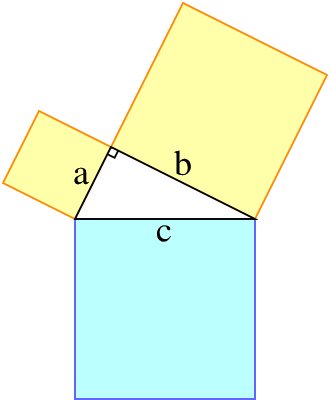The Pythagorean Theorem relates the 3 side lengths a, b, and c of a right triangle (c is the hypotenuse, or longest side) by the equation a2 + b2 = c2.

The Pythagorean Theorem cannot be used by itself to find angles.  However, in a right triangle, we can use it to find the 3rd side length of a triangle and then use trig functions (sine, cosine, or tangent) to solve for the angles.

The Pythagorean Theorem only works for right triangles.  However, the Law of Cosines is the more general rule to find angles and for non-right triangles (more on this later).

There are some common Pythagorean Triples you should know about, which are summarized in the following table.

Note that the Pythagorean Triple {6, 8, 10} is made up of multiples of the Pythagorean Triple {3, 4, 5} (every number in the set is doubled).

Likewise, the Pythagorean Triple {9, 12, 15} is made up of multiples of the Pythagorean Triple {3, 4, 5} (every number in the set is tripled).

In general, if the set {a, b, c} is a Pythagorean Triple, and k is a positive number, then the set {ka, kb, kc} is also a Pythagorean Triple.  We can prove this as follows.

Assume that {a, b, c} is a Pythagorean Triple.  Then:

• (ka)2 + (kb)2
• =k2a2 + k2b2
• =k2(a2 + b2)
• =k2(c2)  [a2 + b2 = c2, since {a, b, c} is a Pythagorean Triple]
• =(kc)2

Since (ka)2 + (kb)2 = (kc)2, then {ka, kb, kc} is a Pythagorean Triple.

### When To Use The Pythagorean Theorem

You should use the Pythagorean Theorem when you know the lengths of 2 sides of a right triangle and you want to find the length of the third side.

There are two separate cases, with two different equations you can use:

• Case 1: You know the length of the two legs, but not the hypotenuse.  In that case, you can solve the Pythagorean Theorem equation for c to get c = √(a2 + b2).
• Case 2: You know the length of the hypotenuse c and one of the legs b, but not the other leg a.  In that case, you can solve the Pythagorean Theorem equation for a to get a = √(c2 – b2).

### How To Use The Pythagorean Theorem

Let’s take a look at some examples of how to use the Pythagorean Theorem.

#### Example 1: How To Use The Pythagorean Theorem To Find The Hypotenuse

Let’s say that we have the following right triangle: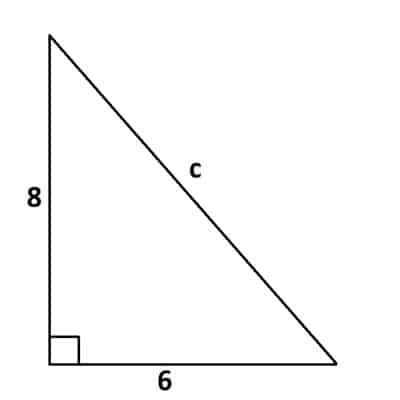This right triangle is missing the length of the hypotenuse, c. We can solve for c in the Pythagorean Theorem to find the length.

To find the hypotenuse, we solve the Pythagorean Theorem for c as follows:

• a2 + b2 = c2
• 62 + 82 = c2
• 36 + 64 = c2
• 100 = c2
• 10 = c

So, the hypotenuse has a length of c = 10.

#### Example 2: How To Use The Pythagorean Theorem To Find The Hypotenuse

Let’s say that we have the following right triangle: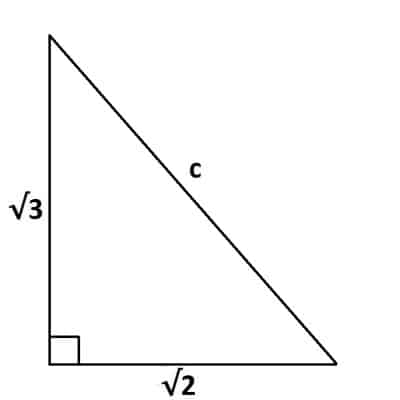This right triangle is missing the length of the hypotenuse, c. We can solve for c in the Pythagorean Theorem to find the length.

To find the hypotenuse, we solve the Pythagorean Theorem for c as follows:

• a2 + b2 = c2
• (√3)2 + (√2)2 = c2
• 3 + 2 = c2
• 5 = c2
• √5 = c

So, the hypotenuse has a length of c = √5.

#### Example 3: How To Use The Pythagorean Theorem To Find A Leg

Let’s say that we have the following right triangle: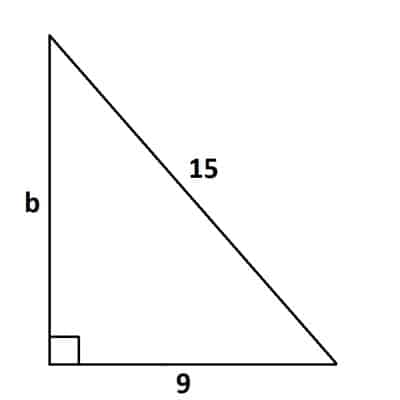This right triangle is missing the length of the leg b. We can solve for b in the Pythagorean Theorem to find the length.

To find the leg, we solve the Pythagorean Theorem for b as follows:

• a2 + b2 = c2
• 92 + b2 = 152
• 81 + b2 = 225
• b2 = 144
• b = 12

So, the leg has a length of b = 12. You may recognize this Pythagorean Triple, {9, 12, 15} from the table earlier in this article – this triple is a multiple of the triple {3, 4, 5}.

#### Example 4: How To Use The Pythagorean Theorem To Find A Leg

Let’s say that we have the following right triangle: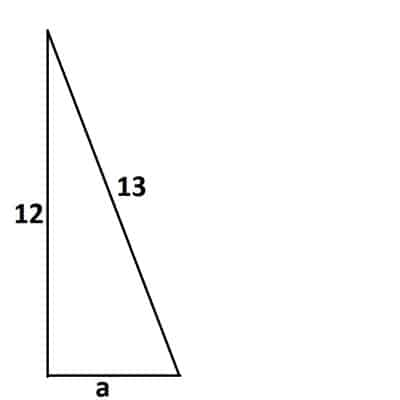This right triangle is missing the length of the leg a. We can solve for a in the Pythagorean Theorem to find the length.

To find the leg, we solve the Pythagorean Theorem for b as follows:

• a2 + b2 = c2
• a2 + 122 = 132
• a2 + 144 = 169
• a2 = 25
• a = 5

So, the leg has a length of a = 5. You may recognize this Pythagorean Triple, {5, 12, 13} from the table earlier in this article.

### Can The Pythagorean Theorem Be Used To Find Angles?

The Pythagorean Theorem cannot be used by itself to find angles.  The reason is that there is no angle involved in the equation for the Pythagorean Theorem: it only relates the side lengths a, b, and c of a right triangle.

However, we can use the Pythagorean Theorem to find the 3rd side length of a right triangle and then use a trig function (sine, cosine, or tangent) to find the angles in the right triangle.

In fact, once we find one of the non-right angles, we automatically know the other one.  If the angles in a right triangle are X, Y, and 90, then they add up to 180:

• X + Y + 90 = 180
• X + Y = 90
• Y = 90 – X

So, if we know the measure of angle X in a right triangle, then we know the measure of Y: 90 – X.

### Can The Pythagorean Theorem Be Applied To Every Triangle?

The Pythagorean Theorem cannot be applied to every triangle.  It only applies to a right triangle (one with a 90 degree angle).

The Pythagorean Theorem does not apply to acute triangles (triangles with 3 acute angles, all less than 90 degrees) or obtuse triangles (triangles with an obtuse angle, greater than 90 degrees).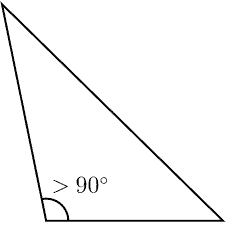The Pythagorean Theorem does not apply to obtuse triangles, which have an angle greater than 90 degrees (and thus no 90 degree angle).

The Law of Cosines relates the side lengths and angles of a triangle, using the cosine trigonometric function.

Assume that a triangle has side lengths a, b, and c, with angles A, B, and C.  Side a is opposite angle A, side b is opposite angle B, and side c is opposite angle C, as shown below: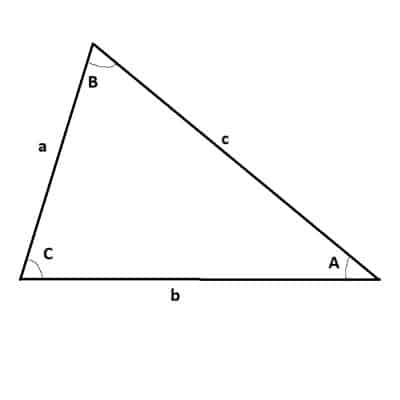For the Law of Cosines, we label the side lengths as a, b, c, with corresponding angles A, B, C (angle A is across from side a, and so on).

The Law of Cosines formula tells us that:

• a2 + b2 – 2abcos(C) = c2

We can also take the square root of both sides to solve for the side length c, which gives us the equation:

• c = √( a2 + b2 – 2abcos(C))

We can also solve for the side length a or b to get the equations:

• a = √(c2 – b2 + 2abcos(C))
• b = √(c2 – a2 + 2abcos(C))

We can even derive the Pythagorean Theorem from the Law of Cosines as follows.

When we have a right triangle, let the right angle (90 degrees) be angle C in the Law of Cosines.  Then we can plug in C = 90 and simplify to get:

• a2 + b2 – 2abcos(C) = c2
• a2 + b2 – 2abcos(90) = c2  [use C = 90 for a right triangle]
• a2 + b2 – 2ab*0 = c2  [since cos(*90) = 0]
• a2 + b2 – 0 = c2
• a2 + b2 = c2

This is the same as the Pythagorean Theorem!  So, the Pythagorean Theorem is a special case of the Law of Cosines in the case when C = 90 degrees (a right triangle).

### Can You Use The Pythagorean Theorem On A Square?

We cannot directly use the Pythagorean Theorem on a square, since the theorem applies to right triangles.  However, we can draw a diagonal for the square to create two identical right isosceles triangles, as shown in the picture below.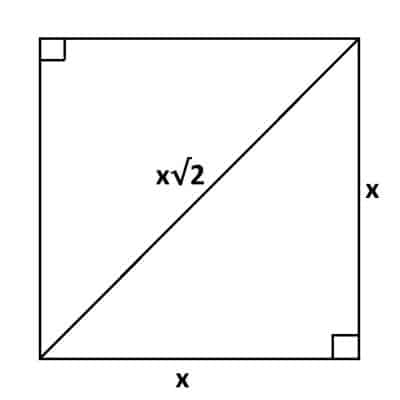We can draw a diagonal to split a square into two equal right isosceles triangles. The length of the diagonal of the square is equal to the length of the hypotenuse of the right triangle.

If the side length of the square is x, then both bases of the right isosceles triangle have a length of x.  Using the Pythagorean Theorem, we can solve for the length of the hypotenuse:

• a2 + b2 = c2
• x2 + x2 = c2  [since both legs have a length of x]
• 2x2 = c2
•  x√2 = c

So, the length of the hypotenuse c of the right triangle is c = x√2, or about 1.41 times the length of the leg x.  The length of the hypotenuse of the right isosceles triangle is also the length of the diagonal of the square.

## Conclusion

Now you know what the Pythagorean Theorem is, when to use it, and how to apply it.  You also know the answers to some common questions about this useful equation.

You can learn about the Triangle Inequality here.